# DAV Class 5 Maths Chapter 7 Brain Teasers Solutions

The DAV Maths Book Class 5 Solutions and DAV Class 5 Maths Chapter 7 Brain Teasers Solutions of Multiplication and Division of Decimal Numbers offer comprehensive answers to textbook questions.

## DAV Class 5 Maths Ch 7 Brain Teasers Solutions

Question 1.
(a) 4562 ÷ _____ = 4.562
(i) 10
(ii) 100
(iii) 1000
(iv) 10000
Solution:
As the decimal point moves two places toward the left we divide it by 100.
So, option (ii) is correct.

(b) 2.5 × 1000 × .1 = _____
(i) 25000
(ii) 2500
(iii) 250
(iv) 2.500
Solution:
2.5 × 1000 × .1
= $$\frac{25}{10}$$ × 1000 × $$\frac{1}{10}$$
= 250
So, option (iii) is correct.(c) The product of 0.2, 0.02, 0.002 is-
(i) 0.08
(ii) 0.008
(iii) 0.000008
(iv) 0.00008
Solution:
0.2 × 0.02 × 0.002 = 0.000008
(decimal moves 6 places left)
So, option (iii) is correct.

(d) The decimal form of $$\frac{9}{25}$$ is-
(i) 0.36
(ii) 3.6
(iii) 3.06
(iv) 36
Solution:So, option (i) is correct.

Question 2.
Find the product of the following:
(a) 8.05 × 16
(b) 14.89 × 2.6
(c) 7.8 × 0.005
Solution:
(a)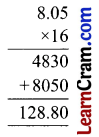(b)(c) 7.8 × 0.005
78 × 5 = 390
7.8 × 0.005 = 0.0390
(Put decimal point after counting the decimal places in multiplicand and multiplier).Question 3.
Find the quotient for the following division questions.
(a) 1.5 ÷ 12
Solution:
1.5 ÷ 12
Dividend = 1.5
Divisor = 12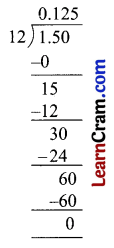Q = 0.125

(b) 122.455 ÷ 0.05
Solution:
122.455 ÷ 0.05
Dividend = 122.455
Divisor = 0.05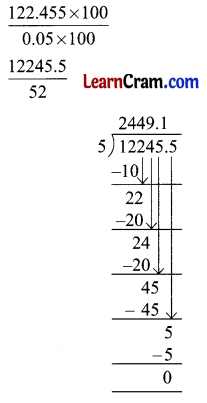Q = 2449.1

(c) 3622 ÷ 45.275
Solution:
3622 ÷ 45.275
Dividend = 3622
Divisor = 45.275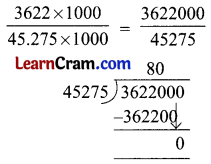Q = 80Question 4.
Convert into a decimal number.
(a) $$\frac{3}{20}$$
Solution:
$$\frac{3}{20}$$(b) 5$$\frac{1}{50}$$
Solution:
5$$\frac{1}{50}$$ = $$\frac{251}{50}$$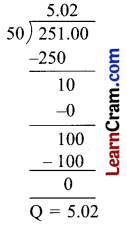(c) 7$$\frac{3}{8}$$
Solution:
7$$\frac{3}{8}$$ = $$\frac{59}{8}$$Question 5.
If 504 ÷ 12 = 42 and 504 × 12 = 6048, find the value of:
(a) 5.04 × 12
Solution:
5.04 × 12 = 60.48
(Place decimal point 2 places to the left)

(b) 50.4 × 12
Solution:
50.4 × 12 = 604.8
(Place decimal point 1 place to the left)

(c) 0.504 × 0.12
Solution:
0.504 × 0.12 = .06048
(Place decimal point five places to the left)

(d) 5.04 ÷ 12
Solution:
5.04 ÷ 12 = 0.42
(Place decimal point two places to the left)(e) 50.4 ÷ 12
Solution:
50.4 ÷ 12 = 4.2
(Place decimal point one place to the left)

(f) 0.504 ÷ 12
Solution:
0.504 ÷ 12 = .042
(Place decimal point three places to the left)

Question 6.
Fill in the boxes.
(a)Solution:
3.5 × 10 = 35
(decimal point moves 1 place right side)

(b)Solution:
1.5 × 1000 = 1500
(decimal point moves 3 places right side)

(c)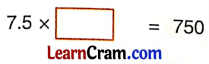Solution:
7.5 × 100 = 750
(decimal point moves 2 places right side)

(d)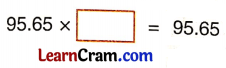Solution:
95.65 × 1 = 95.65
(product remains the same)

(e)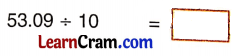Solution:
53.09 ÷ 10 = 5.309
(decimal moves 1 place left side)(f)Solution:
1.98 ÷ 100 = .0198
(decimal moves 2 places left side)

(g)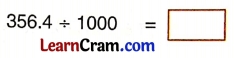Solution:
356.4 ÷ 1000 = 0.3564
(decimal moves 3 places left side)

(h)Solution:
13.8 ÷ 10 = 1.38
(Decimal moves 1 place left side)

(i)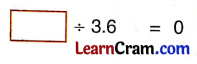Solution:
0 ÷ 3.6 = 0
(0 divided by any decimal number is 0)

(j)Solution:
19.85 × 1 = 19.85
(If a no. multiply by 1, the product is the number itself)

Question 7.
Divide 1010.101 by 1.01
Solution: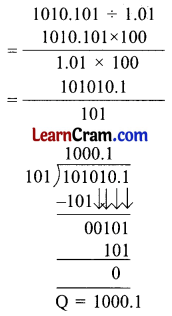Question 8.
3.5 kg toffees are to be distributed among some children if each child has to be given 0.5 kg. toffees. How many children get the toffees?
Solution:
Quantity of toffees to be distributed = 3.5 kg
Quantity to be given to each child = 0.5 kg
No. of children get the toffees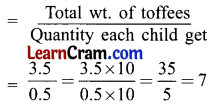7 children got the toffees.

Question 9.
Mr Ajay purchases 3 kg tomatoes at ₹ 15.50 per kilogram and 5.5 kg. potatoes at ₹ 22 per kilogram. Find the total amount spent in all.
Solution:
1 kg tomatoes cost = ₹ 15.50
3 kg tomatoes cost = ₹ 15.50 × 3 = ₹ 46.50
1 kg potatoes cost = ₹ 22
5.5 kg potatoes cost = ₹ 22 × 5.5 = ₹ 121
Total amount spent = Cost of tomatoes + cost of potatoes
= ₹ 46.50 + ₹ 121
= ₹ 167.50

Question 10.
Which of the following has 15 as a quotient?
(a) 0.075 ÷ 0.5
Solution:(b) 0.075 ÷ 0.005
Solution:(c) 0.75 ÷ 5
Solution: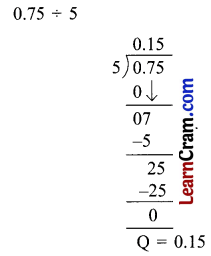Question 1.
Find the product.
(a) 0.012 × 9
Solution:
0.012 × 9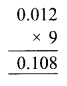(b) 0.56 × 14
Solution:
0.56 × 14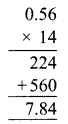(c) 0.76 × 28
Solution:
0.76 × 28(d) 1.001 × 30
Solution:
1.001 × 30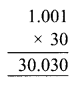(e) 3.01 × 945
Solution:
3.01 × 945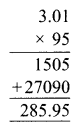Question 2.
Find the product of the following:
(a) 0.012 × 0.01
Solution:
0.012 × 0.01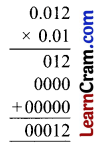(b) 0.75 × 3.4
Solution:
0.75 × 3.4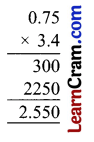(c) 0.170 × 5.6
Solution:
0.170 × 5.6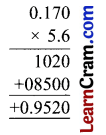(d) 9.378 × 100
Solution:
9.378 × 100(e) 0.11 × 1000
Solution:
0.11 × 1000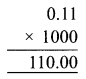(f) 70.16 × 10
Solution:
70.16 × 10Question 3.
Divide the following:
(a) 7.86 ÷ 2
Solution: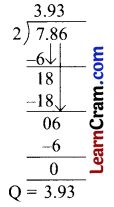(b) 9.085 ÷ 5
Solution: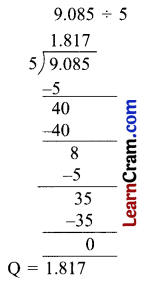(c) 9.1 ÷ 13
Solution:(d) 0.95 ÷ 0.19
Solution:(e) 1.69 ÷ 1.3
Solution:(f) 6.25 ÷ 2.5
Solution: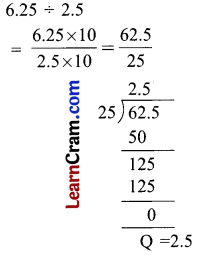Question 4.
Solve the following:
(a) 7.8 ÷ _____ = 1
Solution:
7.8 ÷ 7.8 = 1

(b) 0 ÷ 3.17 = _____
Solution:
0 ÷ 3.17 = 0

(c) 3.78 × 100 = _____
Solution:
3.78 × 100 = 378

(d) 9.376 × 0 = _____
Solution:
9.376 × 0 = 0

(e) 1.019 × _____ = 1.019
Solution:
1.019 × 1 = 1.019

(f) 3.6 × 1.3 = 1.3 × _____
Solution:
3.6 × 1.3 = 1.3 × 3.6

(g) 9.08 ÷ _____ = 1
Solution:
9.08 ÷ 9.08 = 1

(h) 7.39 ÷ 1 = _____
Solution:
7.39 ÷ 1 = 7.39Question 5.
Manik-bought oranges cost ₹ 25 per kg. He bought 3.4 kg oranges. Find the cost of oranges.
Solution:
Cost of 1 kg of oranges, = ₹ 25
Cost of 3.5 kg of oranges= ₹ 25 × 3.5 = ₹ 87.5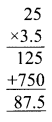The cost of oranges is ₹ 87.5.

Question 6.
Convert into decimals.
(a) $$\frac{1}{2}$$
Solution: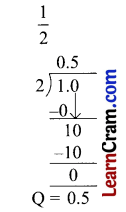(b) $$\frac{3}{8}$$
Solution: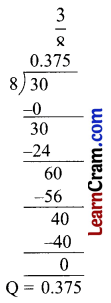(c) 2$$\frac{7}{4}$$
Solution: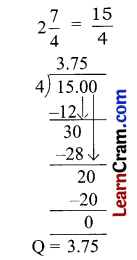(d) 3$$\frac{1}{25}$$
Solution:Question 7.
Rakhi bought 25 packs of plastic colours for ₹ 56.25. Find the cost of 1 pack of plastic colours.
Solution:
Cost of 25 packs = ₹ 56.25
Cost of 1 pack = ₹ 56.25 ÷ 251 pack of plastic colours costs ₹ 2.25.

Question 8.
Complete the following:
(a) 316.32 ÷ _____ = 1
Solution:
316.32 ÷ 316.32 = 1

(b) 27.367 ÷ 1 = _____
Solution:
27.367 ÷ 1 = 27.367

(c) 0 ÷ 317.576 = _____
Solution:
0 ÷ 317.576 = 0

(d) 0.018 × 0 = _____
Solution:
0.018 × 0 = 0

(e) 718.18 × 1 = _____
Solution:
718.18 × 1 = 718.18

Question 9.
Find the product of the following.
(a) 0.8 × 0.08 × 0.008
Solution:
0.8 × 0.08 × 0.008 = 0.000512

(b) 0.02 × 0.001
Solution:
0.02 × 0.001 = 0.00002(c) 18.96 × 13
Solution:
18.96 × 13 = 246.48

(d) 27.36 × 7.6
Solution:
27.36 × 7.6 = 207.936

Question 10.
If 130 ÷ 10 = 13 and 130 × 10 = 1300, find the value of:
(a) 1.30 × 10
Solution:
1.30 × 10 = 13

(b) .013 × 10
Solution:
.013 × 10 = 0.13

(c) 0.13 × 10
Solution:
0.13 × 10 = 1.3

(d) 1.30 ÷ 10
Solution:
1.30 ÷ 10 = 0.13

Question 11.
Fill the grid given below.
(a)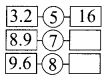Solution: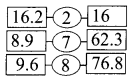(b)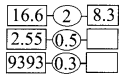Solution: